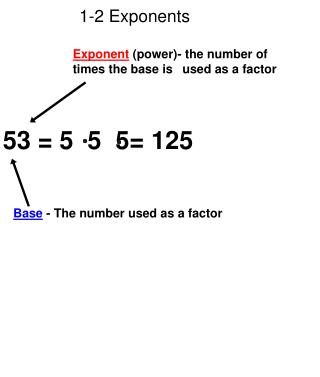DownloadDownload Presentation1-2 Exponents

# 1-2 Exponents

Download Presentation## 1-2 Exponents

- - - - - - - - - - - - - - - - - - - - - - - - - - - E N D - - - - - - - - - - - - - - - - - - - - - - - - - - -
##### Presentation Transcript

1. 1-2 Exponents Exponent (power)- the number of times the base is  used as a factor 53 = 5 5 5= 125 Base - The number used as a factor

2. Special Powers Zero power - Any nonzero number raised  to the zero power is always one (60 = 1) 80 = __________ 100 = __________ 2000 = _________ First power- Any number raised to the first power will always equal itself ( 81 = 8) 71 = __________ 91 = __________ 1001 = _________

3. Write the product using an exponent. 1.) 4 4 4 2.) b b b b b Write the power in words and as repeated multiplication. Then evaluate the power. 3.) 53 4.) 36 Evaluate the expression when x= 8 and x = 0.3 5.) x3  6.) x4

4. 1-3 Solve this Problem 35- 20 5 23 + 8 =

5. Order of Operations When we have many operations we must follow the  correct order of operations. P (Please) Parentheses (grouping symbols) E (Excuse) Exponents M (My) Multiply and D (Dear) Divide LEFT TO RIGHT ORDER A (Aunt) Add and S(Sally) Subtract_ LEFT TO RIGHT ORDER

6. Another way to remember this is the mnemonic device  PEMDAS. 1.) 3 + 144  12 =    2.) 27 - 18 =  3.) (2  = 4.) 2(6+4) 

7. 5.) 72 + 2(8  1)   6.) 6 + 22 2  4 x 2     7.) [(10+2) 4]3  8.) (7 - 2)2 + 34

8. 12 + 3 5 9.) 6 + 3  10.) 3 33 + 7 1 12.) 10 [ 9 – ( 2 2) ] 11.) 3 • 5 – 8 4 + 6